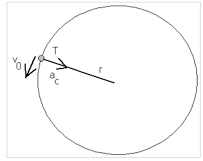# Circular Motion

Circular motion and rotational motion are not the same.

Circular motion is about some external point; rotational motion is about an internal axis.

Rotational motion is motion about an internal axis (the Earth rotates on its axis). Angular quantities are beyond the scope of this course and will not be discussed. AP Physics C uses rotational motion in more depth.   For the diagram below, there are two types of motion going on at the same time…rotational motion (about the sphere’s axis) and translational motion (in a straight line).Circular motion is motion following a circular (curved) path.

When an object has a circular motion, the distance moved is along the circumference of the object’s path.

We have already established that in order to follow a curved path, an object must have 2 separate motions (which can be represented by vectors drawn perpendicular to each other). See the diagram below to illustrate the velocity vector (v) and the tension vector (T), which will represent the centripetal force that is directed towards the center of the circle. You will also see the centripetal acceleration vector (ac), which is also directed toward the center of the circle. Notice that v is always perpendicular to ac and Fc.It has been established that in order to change its motion (such as changing directions to follow a curved path), an object must be undergoing acceleration. And that acceleration must be caused by some net (unbalanced) force.

The acceleration that produces circular motion is called centripetal acceleration . The force that produces the centripetal acceleration is called a centripetal force. Centripetal means toward the center. Centripetal force can be due to one of three physical forces – tension, gravitational force, or friction – to be discussed in more detail on the next pages. Centripetal force is NEVER placed on a free-body diagram. But the other three forces would be placed instead!

Fc = mv2/r

(source)